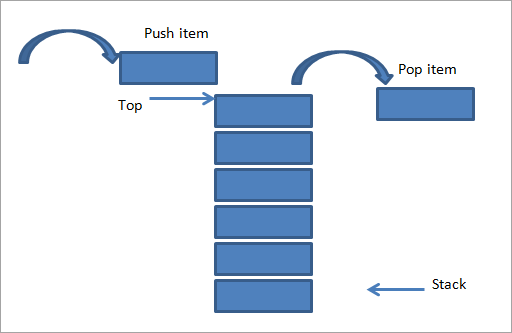# Stack in Data Structure

### What is Stack?

A stack is a non-primitive linear data structure. it is an ordered list in which the addition of a new data item and deletion of the already existing data item is done from only one end known as the top of the stack (TOS). The element which is added in last will be first to be removed and the element which is inserted first will be removed in last. As all the deletion and insertion in a stack is done from the top of the stack, the last added element will be the first to be removed from the stack. That is the reason why stack is also called Last-in-First-out (LIFO).The most frequently accessible element in the stack is the topmost element, whereas the least accessible element is the bottom of the stack. Push and Pop are two operations that are provided for insertion and deletion from the top of the stack respectively.

## Stack Implementation

The stack can be implemented in two ways :

1. Static implementation
2. Dynamic implementation

Static Implementation: – Here array is used to create a stack. it is a simple technique but is not a flexible way of creation, as the size of the stack has to be declared during program design, after that size implementation is not efficient with respect to memory utilization.

Dynamic implementation: – It is also called linked list representation and uses a pointer to implement the stack type of data structure.

### Basics operations on the stack:

Push

Pop

Push operation: – The process of adding new elements to the top of the stack is called push operation.

### Algorithm for PUSH operation

1. Check if the stack is full or not.
2. If the stack is full, then print error of overflow and exit the program.
3. If the stack is not full, then increment the top and add the element.
```PUSH (Stack, Size, TOP, x)
{
if (TOP=Size)
Print stack overflow and exit
TOP = TOP + 1
Stack[TOP] = x
EXIT
}
```

Pop: – The process of deleting an element. from the top of the stack is called POP operation

### Algorithm for POP operation

1. Check if the stack is empty or not.
2. If the stack is empty, then print error of underflow and exit the program.
3. If the stack is not empty, then print the element at the top and decrement the top.
```POP (Stack, Size, TOP)
{
if (TOP==-1)
Print Underflow
X = Stack[TOP]
TOP=TOP-1
Return X and Exit
}
```

Code:

## C++ Code

``````#include <bits/stdc++.h>

using namespace std;

#define MAX 1000

class Stack {
int top;

public:
int arr[MAX]; // Maximum size of Stack

Stack() { top = -1; }

bool push(int value)
{
if (top >= (MAX - 1)) {
cout << "Stack Overflow";
return false;
}
else {
arr[++top] = value;
cout << value << " pushed into stack\n";
return true;
}
}

int pop()
{
if (top < 0) {
cout << "Stack Underflow";
return 0;
}
else {
int val = arr[top--];
return val;
}
}
int peek()
{
if (top < 0) {
cout << "Stack is Empty";
return 0;
}
else {
int val = arr[top];
return val;
}
}

bool isEmpty()
{
return (top < 0);
}
};

int main()
{
class Stack s;
s.push(10);
s.push(20);
s.push(30);
cout << s.pop() << " Popped from stack\n";

cout<<"Elements present in stack : ";
while(!s.isEmpty())
{
cout<<s.peek()<<" ";
s.pop();
}

return 0;
}
``````

Output:

10 pushed into stack
20 pushed into stack
30 pushed into stack
30 Popped from stack
Elements present in stack : 20 10

## Java Code

``````import java.util.*;

class Stack {
static final int MAX = 1000;
static int top;
static int arr[] = new int[MAX]; // Maximum size of Stack

static boolean isEmpty()
{
return (top < 0);
}
Stack()
{
top = -1;
}

static boolean push(int val)
{
if (top >= (MAX - 1)) {
System.out.println("Stack Overflow");
return false;
}
else {
arr[++top] = val;
System.out.println(val + " pushed into stack");
return true;
}
}

static int pop()
{
if (top < 0) {
System.out.println("Stack Underflow");
return 0;
}
else {
int val = arr[top--];
return val;
}
}

static int peek()
{
if (top < 0) {
System.out.println("Stack Underflow");
return 0;
}
else {
int val = arr[top];
return val;
}
}

static void print(){
for(int i = top;i>-1;i--){
System.out.print(" "+ arr[i]);
}
}
}

// Driver code
class TUF {
public static void main(String args[])
{
Stack s = new Stack();
s.push(10);
s.push(20);
s.push(30);
System.out.println(s.pop() + " Popped from stack");
System.out.println("Top element is :" + s.peek());
System.out.print("Elements present in stack :");
s.print();
}
}
``````

Output:

10 pushed into stack
20 pushed into stack
30 pushed into stack
30 Popped from stack
Top element is :20
Elements present in stack : 20 10

### Analysis of Stack Operations:

Below mentioned are the time complexities for various operations that can be performed on the Stack data structure.

• Push Operation : O(1)
• Pop Operation : O(1)
• Top Operation : O(1)
• Search Operation : O(n)

Special thanks to Swarnavo Mondal for contributing to this article on takeUforward. If you also wish to share your knowledge with the takeUforward fam, please check out this article